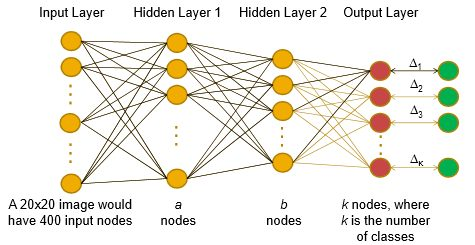An artificial neuron or perceptron takes several inputs and performs a weighted summation to produce an output. The weight of the perceptron is determined during the training process and is based on the training data. The following is a diagram of the perceptron:Figure 7: An example of 4-layer neural network. 1 input layer, 2 hidden layers, and an output layer. Figure from Ptucha

The inputs are weighted and summed as shown in the preceding image. The sum is then passed through a unit step function, in this case, for a binary classification problem. A perceptron can only learn simple functions by learning the weights from examples. The process of learning the weights is called training. The training on a perceptron can be done through gradient-based methods which are explained in a later section. The output of the perceptron can be passed through an activation function or transfer function, which will be explained in the next section.